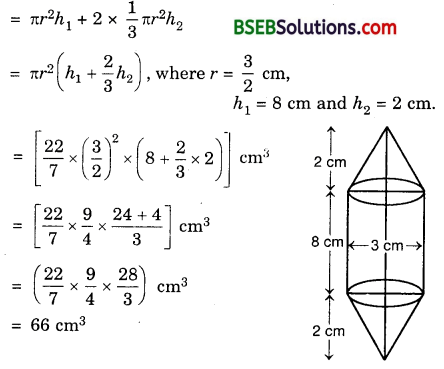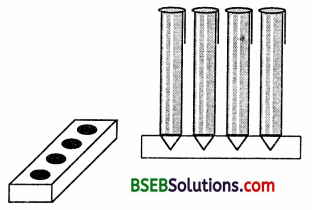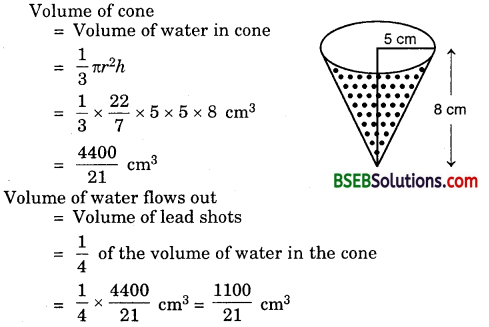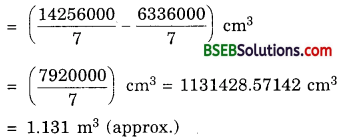# HSSlive: Plus One & Plus Two Notes & Solutions for Kerala State Board

## BSEB Class 10 Maths Chapter 13 Surface Areas and Volumes Ex 13.2 Textbook Solutions PDF: Download Bihar Board STD 10th Maths Chapter 13 Surface Areas and Volumes Ex 13.2 Book AnswersBSEB Class 10 Maths Chapter 13 Surface Areas and Volumes Ex 13.2 Textbook Solutions PDF: Download Bihar Board STD 10th Maths Chapter 13 Surface Areas and Volumes Ex 13.2 Book Answers

BSEB Class 10th Maths Chapter 13 Surface Areas and Volumes Ex 13.2 Textbooks Solutions and answers for students are now available in pdf format. Bihar Board Class 10th Maths Chapter 13 Surface Areas and Volumes Ex 13.2 Book answers and solutions are one of the most important study materials for any student. The Bihar Board Class 10th Maths Chapter 13 Surface Areas and Volumes Ex 13.2 books are published by the Bihar Board Publishers. These Bihar Board Class 10th Maths Chapter 13 Surface Areas and Volumes Ex 13.2 textbooks are prepared by a group of expert faculty members. Students can download these BSEB STD 10th Maths Chapter 13 Surface Areas and Volumes Ex 13.2 book solutions pdf online from this page.

## Bihar Board Class 10th Maths Chapter 13 Surface Areas and Volumes Ex 13.2 Books Solutions

 Board BSEB Materials Textbook Solutions/Guide Format DOC/PDF Class 10th Subject Maths Chapter 13 Surface Areas and Volumes Ex 13.2 Chapters All Provider Hsslive

## How to download Bihar Board Class 10th Maths Chapter 13 Surface Areas and Volumes Ex 13.2 Textbook Solutions Answers PDF Online?

2. Click on the Bihar Board Class 10th Maths Chapter 13 Surface Areas and Volumes Ex 13.2 Answers.
3. Look for your Bihar Board STD 10th Maths Chapter 13 Surface Areas and Volumes Ex 13.2 Textbooks PDF.
4. Now download or read the Bihar Board Class 10th Maths Chapter 13 Surface Areas and Volumes Ex 13.2 Textbook Solutions for PDF Free.

## BSEB Class 10th Maths Chapter 13 Surface Areas and Volumes Ex 13.2 Textbooks Solutions with Answer PDF Download

Find below the list of all BSEB Class 10th Maths Chapter 13 Surface Areas and Volumes Ex 13.2 Textbook Solutions for PDF’s for you to download and prepare for the upcoming exams:

## BSEB Bihar Board Class 10th Maths Solutions Chapter 13 Surface Areas and Volumes Ex 13.2

Question 1.
A solid is in the shape of a cone standing on a hemisphere with both their radii being equal to 1 cm and the height of the cone is equal to its radius. Find the volume of the solid in terms of n.
Solution:
Volume of the solid = Volume of the cone + Volume of the hemisphereQuestion 2.
Rachel, an engineering student was asked to make a model shaped like a cylinder with two cones attached at its two ends by using thin aluminium sheet. The diameter of the model is 3 cm and its length is 12 cm. If each cone has a height of 2 cm, find the volume of air contained in the model the Rachel made. (Assume the outer and inner dimensions of the model to be nearly the same).
Solution:
Volume of the air contained in the model = Volume of the cylindrical portion of the model + Volume of its two conical endsQuestion 3.
A gulab jamun, contains sugar syrup up to about 30% of its volume. Find approximately how much syrup would be found in 45 gulab jamuns, each shaped like a cylinder with two hemispherical ends with length 5 cm and diameter is 2.8 cm (see figure).[Annual Paper (Delhi) 2008]
Solution:
Volume of the gulab jamun = Volume of the cylindrical portion + Volume of the two hemispherical endsVolume of 45 gulab jamuns
= 45 x 227 x 1.96 x 12.23 cm³Quantity of syrup in gulab jamuns = 30% of their volume
= 30100 x 45 x 227 x 1.96 x 12.23 cm³
= 9×11×1.96×12.27 cm³
= 338.184 cm³ = 338 cm³ (approx.)

Question 4.
A pen stand made of wood is in the shape of a cuboid with four conical depressions to hold pens. The dimensions of the cuboid are 15 cm by 10 cm by 3.5 cm. The radius of each of the depressions is 0.5 cm and the depth is 1.4 cm. Find the volume of wood in the entire stand (see figure).Solution:
Volume of wood in the entire stand = Volume of the cuboid – 4 x Volume of a conical depression
= lbh – 4 x 13 πr²H, where l, b, h are dimensions of cuboid, r is radius of the cone and H is its depth
= 15 x 10 x 3.5 cm³ – 4 x 13 x 227 x 0.5 x 0.5 x 1.4 cm³
= (525 – 1.47) cm³ = 523.53 cm³.

Question 5.
A vessel is in the form of an inverted cone. Its height is 8 cm and the radius of its top, which is open, is 5 cm. It is filled with water up to the brim. When lead shots, each of which is a sphere of radius 0.5 cm are dropped into the vessel, one-fourth of the water flows out. Find the number of lead shots dropped in the vessel.
Solution:
Height of the conical vessel h = 8 cm.
Its radius r = 5 cm.Volume of one spherical lead shotQuestion 6.
A solid iron pole consists of a cylindrical height 220 cm and base diameter 24 cm, which is surmounted by another cylinder of height 60 cm and radius 8 cm. Find the mass of the pole, given that 1 cm³ of iron has approximately 8 g mass. (Use π = 3.14)
Solution:
Volume of the solid iron pole = Volume of the base cylindrical portion + Volume of the other cylindrical portion
= πr1² + πr2² h2
= (3.14 x (12)² x 220 + 3.14 x (8)² x 60) cm³
= (3.14 x 144 x 220 + 3.14 x 64 x 60) cm³
= (99475.2 + 12057.6) cm³
= 111532.8 cm³
Hence, the mass of the pole= (111532.8 x 8) grams
= (111532.8×81000) kg
= 892.26 kg.

Question 7.
A solid consisting of a right circular cone of height 120 cm and radius 60 cm standing on a hemisphere of radius 60 cm is placed upright in a right circular cylinder full of water such that it touches the bottom. Find the volume of water left in the cylinder, if the radius of the cylinder is 60 cm and its height is 180 cm.
Solution:
Volume of the cylinder = πr²hVolume of water left in the cylinder = Volume of the cylinder – Volume of the solidQuestion 8.
A spherical glass vessel has a cylindrical neck 8 cm long, 2 cm in diameter; the diameter of the spherical part is 8.5 cm. By measuring the amount of water it holds, a child finds its volume to be 345 cm³. Check whether she is correct, taking the above as the inside measurements and n = 3.14.
Solution:
Volume of spherical vessel = Volume of cylindrical part + Volume of spherical part= π(1)²(8) + 43 π(4.25)³
= 3.14[8 + 43 x 76.765625] cm³
= 3.14[8 + 102.353] cm³
= 3.14 x 110.353 = 346.51 cm³

## Bihar Board Class 10th Maths Chapter 13 Surface Areas and Volumes Ex 13.2 Textbooks for Exam Preparations

Bihar Board Class 10th Maths Chapter 13 Surface Areas and Volumes Ex 13.2 Textbook Solutions can be of great help in your Bihar Board Class 10th Maths Chapter 13 Surface Areas and Volumes Ex 13.2 exam preparation. The BSEB STD 10th Maths Chapter 13 Surface Areas and Volumes Ex 13.2 Textbooks study material, used with the English medium textbooks, can help you complete the entire Class 10th Maths Chapter 13 Surface Areas and Volumes Ex 13.2 Books State Board syllabus with maximum efficiency.

## FAQs Regarding Bihar Board Class 10th Maths Chapter 13 Surface Areas and Volumes Ex 13.2 Textbook Solutions

#### How to get BSEB Class 10th Maths Chapter 13 Surface Areas and Volumes Ex 13.2 Textbook Answers??

Students can download the Bihar Board Class 10 Maths Chapter 13 Surface Areas and Volumes Ex 13.2 Answers PDF from the links provided above.

#### Can we get a Bihar Board Book PDF for all Classes?

Yes you can get Bihar Board Text Book PDF for all classes using the links provided in the above article.

## Important Terms

Bihar Board Class 10th Maths Chapter 13 Surface Areas and Volumes Ex 13.2, BSEB Class 10th Maths Chapter 13 Surface Areas and Volumes Ex 13.2 Textbooks, Bihar Board Class 10th Maths Chapter 13 Surface Areas and Volumes Ex 13.2, Bihar Board Class 10th Maths Chapter 13 Surface Areas and Volumes Ex 13.2 Textbook solutions, BSEB Class 10th Maths Chapter 13 Surface Areas and Volumes Ex 13.2 Textbooks Solutions, Bihar Board STD 10th Maths Chapter 13 Surface Areas and Volumes Ex 13.2, BSEB STD 10th Maths Chapter 13 Surface Areas and Volumes Ex 13.2 Textbooks, Bihar Board STD 10th Maths Chapter 13 Surface Areas and Volumes Ex 13.2, Bihar Board STD 10th Maths Chapter 13 Surface Areas and Volumes Ex 13.2 Textbook solutions, BSEB STD 10th Maths Chapter 13 Surface Areas and Volumes Ex 13.2 Textbooks Solutions,
Share: